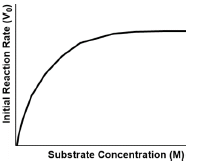Clutch Prep is now a part of Pearson
Ch. 6 - Enzymes and Enzyme KineticsWorksheetSee all chapters

# Initial Velocity

See all sections
Sections
Enzymes
Enzyme-Substrate Complex
Lock and Key Vs. Induced Fit Models
Optimal Enzyme Conditions
Activation Energy
Types of Enzymes
Cofactor
Catalysis
Electrostatic and Metal Ion Catalysis
Covalent Catalysis
Reaction Rate
Enzyme Kinetics
Rate Constants and Rate Law
Reaction Orders
Rate Constant Units
Initial Velocity
Vmax Enzyme
Km Enzyme
Michaelis-Menten Assumptions
Michaelis-Menten Equation
Lineweaver-Burk Plot
Michaelis-Menten vs. Lineweaver-Burk Plots
Shifting Lineweaver-Burk Plots
Calculating Vmax
Calculating Km
Kcat
Specificity Constant

Concept #1: Initial Velocity

Concept #2: Enzyme Kinetics Plot (V0 vs [S])

Practice: Why is the initial velocity (V0) the best chance a reaction has at approaching its maximum velocity (Vmax)?

Practice: Calculate the initial reaction rate for A → B, given that [A] i = 9.6 M, [B] i = 0 & [A] f after 0.01 μsec = 9.14 mM.

Practice: In the graph below, why does the curve have a steep incline at first that gradually declines to a horizontal line?